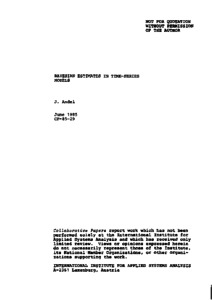Bayesian Estimates in Time-Series Models

Andel, J. (1985). Bayesian Estimates in Time-Series Models. IIASA Collaborative Paper. IIASA, Laxenburg, Austria: CP-85-029Preview
Text
CP-85-029.pdf

Abstract

Autoregressive (AR), moving-average (MA), and autoregressive- moving average (ARMA) models are very popular in time-series analysis. Many problems of estimating their parameters and testing hypotheses are only solved asymptotically. The derivation of asymptotic results is usually not easy. An alternative approach to such problems is the Bayesian approach. It is assumed that the parameters of the models are random variables. There are theorems ensuring that under general assumptions the asymptotic posterior distribution does not depend on the prior distribution. As the derivation of the results is usually easier in the Bayesian approach, we can use this procedure particularly for the statistical analysis of more complicated models.

Item Type: Monograph (IIASA Collaborative Paper) Economic Structural Change Program (ECO) IIASA Import 15 Jan 2016 01:56 27 Aug 2021 17:12 http://pure.iiasa.ac.at/2719View Item